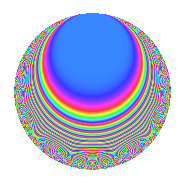Properties

 Label 618.2.aLevel 618 Weight 2 Character orbit a Rep. character $$\chi_{618}(1,\cdot)$$ Character field $$\Q$$ Dimension 17 Newforms 11 Sturm bound 208 Trace bound 5

Related objects

Defining parameters

 Level: $$N$$ = $$618 = 2 \cdot 3 \cdot 103$$ Weight: $$k$$ = $$2$$ Character orbit: $$[\chi]$$ = 618.a (trivial) Character field: $$\Q$$ Newforms: $$11$$ Sturm bound: $$208$$ Trace bound: $$5$$ Distinguishing $$T_p$$: $$5$$

Dimensions

The following table gives the dimensions of various subspaces of $$M_{2}(\Gamma_0(618))$$.

Total New Old
Modular forms 108 17 91
Cusp forms 101 17 84
Eisenstein series 7 0 7

The following table gives the dimensions of the cuspidal new subspaces with specified eigenvalues for the Atkin-Lehner operators and the Fricke involution.

$$2$$$$3$$$$103$$FrickeDim.
$$+$$$$+$$$$+$$$$+$$$$2$$
$$+$$$$+$$$$-$$$$-$$$$2$$
$$+$$$$-$$$$+$$$$-$$$$2$$
$$+$$$$-$$$$-$$$$+$$$$2$$
$$-$$$$+$$$$+$$$$-$$$$4$$
$$-$$$$+$$$$-$$$$+$$$$1$$
$$-$$$$-$$$$+$$$$+$$$$1$$
$$-$$$$-$$$$-$$$$-$$$$3$$
Plus space$$+$$$$6$$
Minus space$$-$$$$11$$

Trace form

 $$17q$$ $$\mathstrut +\mathstrut q^{2}$$ $$\mathstrut -\mathstrut q^{3}$$ $$\mathstrut +\mathstrut 17q^{4}$$ $$\mathstrut +\mathstrut 6q^{5}$$ $$\mathstrut -\mathstrut q^{6}$$ $$\mathstrut +\mathstrut q^{8}$$ $$\mathstrut +\mathstrut 17q^{9}$$ $$\mathstrut +\mathstrut O(q^{10})$$ $$17q$$ $$\mathstrut +\mathstrut q^{2}$$ $$\mathstrut -\mathstrut q^{3}$$ $$\mathstrut +\mathstrut 17q^{4}$$ $$\mathstrut +\mathstrut 6q^{5}$$ $$\mathstrut -\mathstrut q^{6}$$ $$\mathstrut +\mathstrut q^{8}$$ $$\mathstrut +\mathstrut 17q^{9}$$ $$\mathstrut -\mathstrut 2q^{10}$$ $$\mathstrut -\mathstrut 4q^{11}$$ $$\mathstrut -\mathstrut q^{12}$$ $$\mathstrut -\mathstrut 6q^{13}$$ $$\mathstrut -\mathstrut 6q^{15}$$ $$\mathstrut +\mathstrut 17q^{16}$$ $$\mathstrut +\mathstrut 2q^{17}$$ $$\mathstrut +\mathstrut q^{18}$$ $$\mathstrut -\mathstrut 16q^{19}$$ $$\mathstrut +\mathstrut 6q^{20}$$ $$\mathstrut -\mathstrut 8q^{21}$$ $$\mathstrut -\mathstrut 4q^{22}$$ $$\mathstrut -\mathstrut q^{24}$$ $$\mathstrut +\mathstrut 19q^{25}$$ $$\mathstrut -\mathstrut 2q^{26}$$ $$\mathstrut -\mathstrut q^{27}$$ $$\mathstrut +\mathstrut 6q^{29}$$ $$\mathstrut -\mathstrut 2q^{30}$$ $$\mathstrut -\mathstrut 24q^{31}$$ $$\mathstrut +\mathstrut q^{32}$$ $$\mathstrut -\mathstrut 8q^{33}$$ $$\mathstrut +\mathstrut 18q^{34}$$ $$\mathstrut +\mathstrut 24q^{35}$$ $$\mathstrut +\mathstrut 17q^{36}$$ $$\mathstrut -\mathstrut 2q^{37}$$ $$\mathstrut +\mathstrut 4q^{38}$$ $$\mathstrut -\mathstrut 6q^{39}$$ $$\mathstrut -\mathstrut 2q^{40}$$ $$\mathstrut +\mathstrut 2q^{41}$$ $$\mathstrut +\mathstrut 4q^{43}$$ $$\mathstrut -\mathstrut 4q^{44}$$ $$\mathstrut +\mathstrut 6q^{45}$$ $$\mathstrut -\mathstrut 8q^{46}$$ $$\mathstrut +\mathstrut 8q^{47}$$ $$\mathstrut -\mathstrut q^{48}$$ $$\mathstrut +\mathstrut 9q^{49}$$ $$\mathstrut +\mathstrut 31q^{50}$$ $$\mathstrut -\mathstrut 2q^{51}$$ $$\mathstrut -\mathstrut 6q^{52}$$ $$\mathstrut -\mathstrut 2q^{53}$$ $$\mathstrut -\mathstrut q^{54}$$ $$\mathstrut +\mathstrut 24q^{55}$$ $$\mathstrut -\mathstrut 4q^{57}$$ $$\mathstrut +\mathstrut 2q^{58}$$ $$\mathstrut -\mathstrut 20q^{59}$$ $$\mathstrut -\mathstrut 6q^{60}$$ $$\mathstrut +\mathstrut 34q^{61}$$ $$\mathstrut +\mathstrut 24q^{62}$$ $$\mathstrut +\mathstrut 17q^{64}$$ $$\mathstrut +\mathstrut 20q^{65}$$ $$\mathstrut +\mathstrut 4q^{66}$$ $$\mathstrut -\mathstrut 20q^{67}$$ $$\mathstrut +\mathstrut 2q^{68}$$ $$\mathstrut +\mathstrut 8q^{69}$$ $$\mathstrut +\mathstrut 8q^{70}$$ $$\mathstrut -\mathstrut 32q^{71}$$ $$\mathstrut +\mathstrut q^{72}$$ $$\mathstrut -\mathstrut 54q^{73}$$ $$\mathstrut -\mathstrut 2q^{74}$$ $$\mathstrut +\mathstrut q^{75}$$ $$\mathstrut -\mathstrut 16q^{76}$$ $$\mathstrut -\mathstrut 16q^{77}$$ $$\mathstrut -\mathstrut 14q^{78}$$ $$\mathstrut -\mathstrut 16q^{79}$$ $$\mathstrut +\mathstrut 6q^{80}$$ $$\mathstrut +\mathstrut 17q^{81}$$ $$\mathstrut +\mathstrut 10q^{82}$$ $$\mathstrut -\mathstrut 28q^{83}$$ $$\mathstrut -\mathstrut 8q^{84}$$ $$\mathstrut -\mathstrut 28q^{85}$$ $$\mathstrut -\mathstrut 4q^{86}$$ $$\mathstrut +\mathstrut 2q^{87}$$ $$\mathstrut -\mathstrut 4q^{88}$$ $$\mathstrut -\mathstrut 30q^{89}$$ $$\mathstrut -\mathstrut 2q^{90}$$ $$\mathstrut +\mathstrut 24q^{91}$$ $$\mathstrut -\mathstrut 28q^{93}$$ $$\mathstrut -\mathstrut 16q^{94}$$ $$\mathstrut -\mathstrut 32q^{95}$$ $$\mathstrut -\mathstrut q^{96}$$ $$\mathstrut +\mathstrut 10q^{97}$$ $$\mathstrut +\mathstrut 9q^{98}$$ $$\mathstrut -\mathstrut 4q^{99}$$ $$\mathstrut +\mathstrut O(q^{100})$$

Decomposition of $$S_{2}^{\mathrm{new}}(\Gamma_0(618))$$ into irreducible Hecke orbits

Label Dim. $$A$$ Field CM Traces A-L signs $q$-expansion
$$a_2$$ $$a_3$$ $$a_5$$ $$a_7$$ 2 3 103
618.2.a.a $$1$$ $$4.935$$ $$\Q$$ None $$-1$$ $$-1$$ $$-1$$ $$-2$$ $$+$$ $$+$$ $$+$$ $$q-q^{2}-q^{3}+q^{4}-q^{5}+q^{6}-2q^{7}+\cdots$$
618.2.a.b $$1$$ $$4.935$$ $$\Q$$ None $$-1$$ $$-1$$ $$2$$ $$-2$$ $$+$$ $$+$$ $$+$$ $$q-q^{2}-q^{3}+q^{4}+2q^{5}+q^{6}-2q^{7}+\cdots$$
618.2.a.c $$1$$ $$4.935$$ $$\Q$$ None $$-1$$ $$1$$ $$-3$$ $$2$$ $$+$$ $$-$$ $$-$$ $$q-q^{2}+q^{3}+q^{4}-3q^{5}-q^{6}+2q^{7}+\cdots$$
618.2.a.d $$1$$ $$4.935$$ $$\Q$$ None $$-1$$ $$1$$ $$0$$ $$-4$$ $$+$$ $$-$$ $$-$$ $$q-q^{2}+q^{3}+q^{4}-q^{6}-4q^{7}-q^{8}+\cdots$$
618.2.a.e $$1$$ $$4.935$$ $$\Q$$ None $$1$$ $$-1$$ $$-2$$ $$-2$$ $$-$$ $$+$$ $$-$$ $$q+q^{2}-q^{3}+q^{4}-2q^{5}-q^{6}-2q^{7}+\cdots$$
618.2.a.f $$1$$ $$4.935$$ $$\Q$$ None $$1$$ $$1$$ $$-4$$ $$-4$$ $$-$$ $$-$$ $$+$$ $$q+q^{2}+q^{3}+q^{4}-4q^{5}+q^{6}-4q^{7}+\cdots$$
618.2.a.g $$1$$ $$4.935$$ $$\Q$$ None $$1$$ $$1$$ $$3$$ $$-2$$ $$-$$ $$-$$ $$-$$ $$q+q^{2}+q^{3}+q^{4}+3q^{5}+q^{6}-2q^{7}+\cdots$$
618.2.a.h $$2$$ $$4.935$$ $$\Q(\sqrt{3})$$ None $$-2$$ $$-2$$ $$2$$ $$6$$ $$+$$ $$+$$ $$-$$ $$q-q^{2}-q^{3}+q^{4}+(1+\beta )q^{5}+q^{6}+\cdots$$
618.2.a.i $$2$$ $$4.935$$ $$\Q(\sqrt{2})$$ None $$-2$$ $$2$$ $$4$$ $$0$$ $$+$$ $$-$$ $$+$$ $$q-q^{2}+q^{3}+q^{4}+(2+\beta )q^{5}-q^{6}+\cdots$$
618.2.a.j $$2$$ $$4.935$$ $$\Q(\sqrt{2})$$ None $$2$$ $$2$$ $$0$$ $$4$$ $$-$$ $$-$$ $$-$$ $$q+q^{2}+q^{3}+q^{4}+\beta q^{5}+q^{6}+(2+\beta )q^{7}+\cdots$$
618.2.a.k $$4$$ $$4.935$$ 4.4.54332.1 None $$4$$ $$-4$$ $$5$$ $$4$$ $$-$$ $$+$$ $$+$$ $$q+q^{2}-q^{3}+q^{4}+(1+\beta _{1})q^{5}-q^{6}+\cdots$$

Decomposition of $$S_{2}^{\mathrm{old}}(\Gamma_0(618))$$ into lower level spaces

$$S_{2}^{\mathrm{old}}(\Gamma_0(618)) \cong$$ $$S_{2}^{\mathrm{new}}(\Gamma_0(103))$$$$^{\oplus 4}$$$$\oplus$$$$S_{2}^{\mathrm{new}}(\Gamma_0(206))$$$$^{\oplus 2}$$$$\oplus$$$$S_{2}^{\mathrm{new}}(\Gamma_0(309))$$$$^{\oplus 2}$$